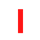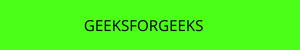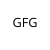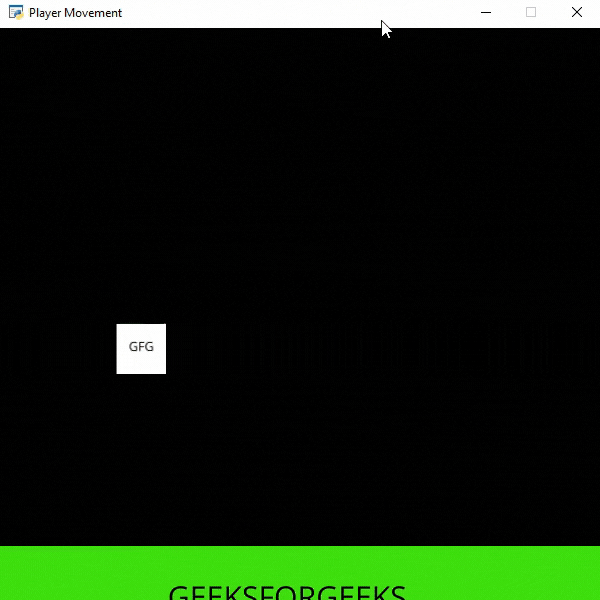Open in App
Not now

• Last Updated : 17 Oct, 2021

In this example, we are going to add bullets to the screen.  For this, we will use some functions:

draw_text(): This function is used to draw text to the screen using Pyglet’s label.

Syntax: arcade.draw_text(text, x, y, color, size, width, align, font_name)

Parameters:

• text: Text we want to display
• x : x coordinate
• y : y coordinate
• color : color of the text
• size : Size of the font
• width : Width of the text
• align : Alignment of the text
• font_name : Name of the font

PhysicsEnginePlatformer(): Simplistic physics engine for use in a platformer.

Parameters:

• player_sprite: sprite of the player
• platforms: The sprites it can’t move through
• gravity: Downward acceleration per frame

### Sprites Used():In the below example, we are going to create a MainGame() class. Inside this class first, we are going to initialize some variables for velocity, scene, bullet sprite, and player’s sprite then we will create 4 functions inside this class.

• on_draw(): Inside this function, we will use our scene on the screen.
• setup(): In this function, we will initialize our scene object then we will load our player and platform’s sprites. After that, we will call the PhysicsEnginePlatformer() function.
• on_update(): In this function, we will update the physics engine, x coordinates of the player’s sprite, and bullet y-coordinate.
• on_key_press() and on_key_release(): In this function, we will change the value of the velocity variable according to the keyboard key that is pressed or released. When the user presses the down arrow key then we will load our bullet sprite.

Below is the implementation:

## Python3

 `# Importing arcade module``import` `arcade`` ` `# Creating MainGame class``class` `MainGame(arcade.Window):``    ``def` `__init__(``self``):``        ``super``().__init__(``600``, ``600``,``                         ``title``=``"Player Movement"``)`` ` `        ``# Initializing a variable to store``        ``# the velocity of the player``        ``self``.vel_x ``=` `0`` ` `        ``# Creating variable for bullet sprite``        ``self``.bullet ``=` `None`` ` `        ``# Creating variable to store player sprite``        ``self``.player ``=` `None`` ` `        ``# Creating variable for our game engine``        ``self``.physics_engine ``=` `None`` ` `    ``# Creating on_draw() function to draw on the screen``    ``def` `on_draw(``self``):``        ``arcade.start_render()`` ` `        ``# Drawing our scene``        ``self``.scene.draw()`` ` `    ``def` `setup(``self``):``         ``# Initialize Scene object``        ``self``.scene ``=` `arcade.Scene()`` ` `        ``# Creating different sprite lists``        ``self``.scene.add_sprite_list(``"Player"``)``        ``self``.scene.add_sprite_list(``"Platforms"``,``                                   ``use_spatial_hash``=``True``)`` ` `        ``# Adding player sprite``        ``self``.player_sprite ``=` `arcade.Sprite(``"Player.png"``, ``1``)`` ` `        ``# Adding coordinates for the center of the sprite``        ``self``.player_sprite.center_x ``=` `64``        ``self``.player_sprite.center_y ``=` `90`` ` `        ``# Adding Sprite in our scene``        ``self``.scene.add_sprite(``"Player"``, ``self``.player_sprite)`` ` `        ``# Adding platform sprite according to level``        ``platform ``=` `arcade.Sprite(f``"Platform.png"``, ``1``)``         ` `        ``# Adding coordinates for the center of the platform``        ``platform.center_x ``=` `300``        ``platform.center_y ``=` `32``        ``self``.scene.add_sprite(``"Platforms"``, platform)`` ` `        ``# Creating Physics engine``        ``self``.physics_engine ``=` `arcade.PhysicsEnginePlatformer(``            ``self``.player_sprite, ``self``.scene.get_sprite_list(``"Platforms"``), ``0.5``        ``)`` ` `    ``# Creating on_update function to``    ``# update the x coordinate``    ``def` `on_update(``self``, delta_time):`` ` `        ``# Changing x coordinate of player``        ``self``.player_sprite.center_x ``+``=` `self``.vel_x ``*` `delta_time``         ` `        ``# Updating the physics engine to move the player``        ``self``.physics_engine.update()`` ` `        ``# If self.bullet has sprites``        ``if` `self``.bullet !``=` `None``:`` ` `            ``# Iterating over every bullet and changing y coordinate``            ``for` `bullet ``in` `self``.scene.get_sprite_list(``"Bullet"``):``                ``bullet.center_y ``+``=` `5`` ` `    ``# Creating function to change the velocity``    ``# when button is pressed``    ``def` `on_key_press(``self``, symbol, modifier):`` ` `        ``# Checking the button pressed``        ``# and changing the value of velocity``        ``if` `symbol ``=``=` `arcade.key.LEFT:``            ``self``.vel_x ``=` `-``300``        ``elif` `symbol ``=``=` `arcade.key.RIGHT:``            ``self``.vel_x ``=` `300``        ``elif` `symbol ``=``=` `arcade.key.UP:``            ``if` `self``.physics_engine.can_jump():``                ``self``.player_sprite.change_y ``=` `15``        ``elif` `symbol ``=``=` `arcade.key.DOWN:``           ` `            ``# Loading bullet sprite``            ``self``.bullet ``=` `arcade.Sprite(``"Bullet.png"``, ``1``)`` ` `            ``# Initializing the starting coordinates``            ``self``.bullet.center_x ``=` `self``.player_sprite.center_x``            ``self``.bullet.bottom ``=` `self``.player_sprite.top`` ` `            ``# Adding the bullet sprite in the scene``            ``self``.scene.add_sprite(``"Bullet"``, ``self``.bullet)`` ` `    ``# Creating function to change the velocity``    ``# when button is released``    ``def` `on_key_release(``self``, symbol, modifier):`` ` `        ``# Checking the button released``        ``# and changing the value of velocity``        ``if` `symbol ``=``=` `arcade.key.LEFT:``            ``self``.vel_x ``=` `0``        ``elif` `symbol ``=``=` `arcade.key.RIGHT:``            ``self``.vel_x ``=` `0`` ` ` ` `# Calling MainGame class``game ``=` `MainGame()``game.setup()``arcade.run()`

Output:My Personal Notes arrow_drop_up